Test: Fourier Series- 1

# Test: Fourier Series- 1

Test Description

## 10 Questions MCQ Test Signals and Systems | Test: Fourier Series- 1

Test: Fourier Series- 1 for Physics 2023 is part of Signals and Systems preparation. The Test: Fourier Series- 1 questions and answers have been prepared according to the Physics exam syllabus.The Test: Fourier Series- 1 MCQs are made for Physics 2023 Exam. Find important definitions, questions, notes, meanings, examples, exercises, MCQs and online tests for Test: Fourier Series- 1 below.
Solutions of Test: Fourier Series- 1 questions in English are available as part of our Signals and Systems for Physics & Test: Fourier Series- 1 solutions in Hindi for Signals and Systems course. Download more important topics, notes, lectures and mock test series for Physics Exam by signing up for free. Attempt Test: Fourier Series- 1 | 10 questions in 30 minutes | Mock test for Physics preparation | Free important questions MCQ to study Signals and Systems for Physics Exam | Download free PDF with solutions
 1 Crore+ students have signed up on EduRev. Have you?
Test: Fourier Series- 1 - Question 1

### The Fourier series expansion of a real periodic signal with fundamental frequency f0 is given by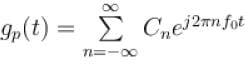It is given that C3 = 3 + 5j then C-3 is Select one:

Detailed Solution for Test: Fourier Series- 1 - Question 1

Given  C3 = 3 + 5j
We know that for real periodic signal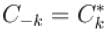So,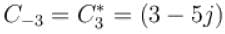The correct answer is: 3 – 5j

Test: Fourier Series- 1 - Question 2

### The Fourier series of an odd periodic function, contains only Select one:

Detailed Solution for Test: Fourier Series- 1 - Question 2

If periodic function is odd the dc term a0 = 0 and also cosine terms (even symmetry)
It contains only sine terms.
The correct answer is: sine terms

Test: Fourier Series- 1 - Question 3

### The trigonometric Fourier series of an even function of time does not have Select one:

Detailed Solution for Test: Fourier Series- 1 - Question 3

For periodic even function, the trigonometric Fourier series does not contain the sine terms (odd functions.)
It has dc term and cosine terms of all harmonic.
The correct answer is: Sine terms

Test: Fourier Series- 1 - Question 4

Trigonometric Fourier series of a periodic time function can have only
Select one:

Detailed Solution for Test: Fourier Series- 1 - Question 4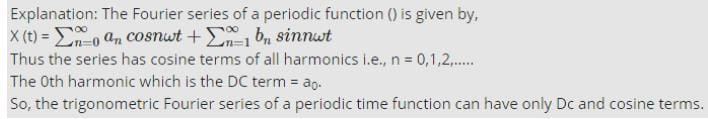Test: Fourier Series- 1 - Question 5

Which of the following cannot be the Fourier series expansion of a periodic signals?
Select one:

Detailed Solution for Test: Fourier Series- 1 - Question 5

→  x(t) = 2cos t + 3cos t is periodic signal with fundamental frequency ω = 1.
→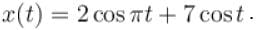The frequency of first term  frequency of 2nd term is ω2 = 1.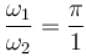is not the rational number
So, x(t) is a periodic or not periodic.
→ x(t) = cos t + 0.5 is a periodic function with
→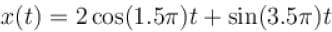first term has frequency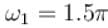2nd term has frequency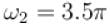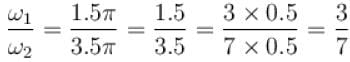So about ratio is rational number x(t) is a periodic signal, with fundamentalfrequency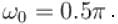Since function in (b) is non periodic. So does not satisfy Dirichlet conditionand cannot be expanded in Fourier series.
The correct answer is: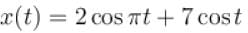Test: Fourier Series- 1 - Question 6

A periodic signal x(t) of period T0 is given by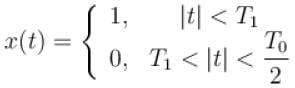The dc component of x(t) is
Select one:

Detailed Solution for Test: Fourier Series- 1 - Question 6

Solution :- The dc component of x(t) is :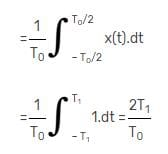Test: Fourier Series- 1 - Question 7

The trigonometric Fourier series of an even function does not have the
Select one:

Detailed Solution for Test: Fourier Series- 1 - Question 7

The trigonometric Fourier series of an even function has cosine terms which are even functions.
It has dc term if its average value is finite and no dc term if average value is zero.
So it does not have sine terms.
The correct answer is: Sine terms

Test: Fourier Series- 1 - Question 8

Choose the function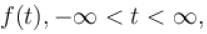for which a Fourier series cannot be defined
Select one:

Detailed Solution for Test: Fourier Series- 1 - Question 8

→  3sin(25t) is periodic ω = 25.
→ 4cos(20t + 3) + 2sin(710t) sum of two periodic function is also periodic function
→ e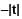sin 25t Due to decaying exponential decaying function it is not periodic.
So Fourier series cannot be defined for it.
→ Constant, Fourier series exists.
Fourier series can’t be defined for option (c).
The correct answer is: exp(–|t|) sin(25t)

Test: Fourier Series- 1 - Question 9

The Fourier series of a real periodic function has only
P. Cosine terms if it is even
Q. Sine terms if it is even
R. Cosine terms if it is odd
S. Sine terms if it is odd
Which of the above statement is correct?
Select one:

Detailed Solution for Test: Fourier Series- 1 - Question 9

The Fourier series for a real periodic function has cosine terms if it is even and sine terms if it is odd.
The correct answer is: P and S

Test: Fourier Series- 1 - Question 10

The Fourier series representation of an impulse train denoted by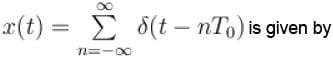Select one:

Detailed Solution for Test: Fourier Series- 1 - Question 10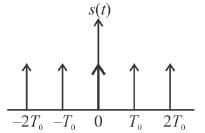The given impulse train s(t) with strength of each impulse as 1 is a periodic function with period T0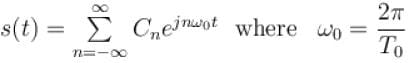where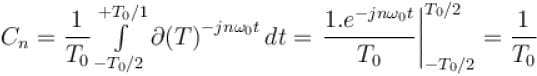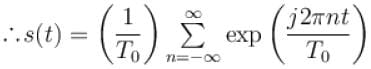The correct answer is: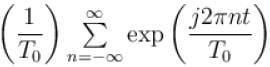## Signals and Systems

40 videos|44 docs|33 tests
Information about Test: Fourier Series- 1 Page
In this test you can find the Exam questions for Test: Fourier Series- 1 solved & explained in the simplest way possible. Besides giving Questions and answers for Test: Fourier Series- 1, EduRev gives you an ample number of Online tests for practice

## Signals and Systems

40 videos|44 docs|33 tests(Scan QR code)